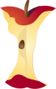Home > Grade Levels > Grade 8 >

## Properties of Integer Exponents#### Aligned To Common Core Standard:

Grade 8 Number System- 8.EE.A.1

### Printable Worksheets And Lessons

• Multiply Exponents Step-by-Step Lesson- Not only that! There are negative exponents. They're on fire while a wake boarder jumps them! Too over the top?

• Guided Lesson - It's all about simplifying exponents. There is also some division and multiplication of exponents along the way too.

• Guided Lesson Explanation - The division problems make really small numbers and multiplication just the opposite.

• Independent Practice - This work sheet has appeared at two National Conferences already. Here I am just thinking that the bat is cute!

• Matching Worksheet - I know that this can be a tough sheet for some kids. They often get choice "c" and "d" confused. Heads up!

• Exponents to Numbers Five Pack - Put the exponents in numerical form and then compare the values of the exponents.

###(Click Here to Upgrade)

#### Homework Sheets

Multiplication and division of exponents followed by exponents of exponents.

#### Practice Worksheets

We follow the same progression in the practice sheets as we did with the homework.

#### Math Skill Quizzes

Once again, we stick with the progression. The quiz problems are standard questions that you will see often.

 Quiz 1 Quiz 2 Quiz 3